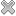Courses / Module

Toggle PrintSTRUCTURAL EQUATION MODELLING

Module code: PS861
Credits: 5
Semester: Year-Long
Department: PSYCHOLOGY
International:Coordinator: Dr Philip Hyland (PSYCHOLOGY)Overview

Introduction to the Latent Variable Modelling approach; Confirmatory Factor Analysis; Basic Structural Equation Modelling; Theory of Change: Mediation analysis in Structural Equation Modelling. The objective of this module is to introduce students to the theory latent variable modelling; how to conduct, interpret, and present Structural Equation Modelling analyses; and how Structural Equation Modelling can be used to determine mechanism of change.Learning OutcomesTeaching & Learning methodsAssessmentTimetable